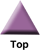### Home

Laboratory
Studies

Recordkeeping, Writing,
& Data Analysis

Laboratory
Methods

Overview
Microscope studies

Flagella experiment
Laboratory math
Blood fractionation
Gel electrophoresis
Protein gel analysis
Mitochondria
Concepts/ theory
Overview
Keeping a lab notebook
Writing research papers
Dimensions & units
Using figures (graphs)
Examples of graphs
Experimental error
Representing error
Applying statistics
Overview
Principles of microscopy

Solutions & dilutions
Protein assays
Spectrophotometry
Fractionation & centrifugation

# The Chi-Squared Goodness-of-Fit Test

A test based upon the Chi-squared distribution is a nonparametric test. Nonparametric tests determine the probability that an observed distribution of data, based upon rankings or distribution into categories of a qualitative nature, is due to chance (sampling error) alone. If you have numbers that appear to follow a normal or t-distribution, then you would want to use a parametric test such as 'Student's' t test to address your question. The chi-square test is very useful, especially when data are not quantitative. It will probably be most effective to explain the process using an example.

## Example: is the distribution of pine trees related to soil type?

You have noticed that pine trees grow well in some parts of the woods, but not others. You speculate that the distribution of pines is related to drainage, that is, that pines prefer a very well-drained soil, while they do poorly in wet areas. You sample soil from evenly spaced plots throughout the forest, two days after a heavy rain. You find that you can describe each plot as belonging to one of three categories of soil: dry (sample falls apart in your hand), loamy (holds shape if you squeeze it, falls apart if you drop it), and wet (muddy - you can squeeze lots of water out, soil tends to run through your fingers).

Now, if soil drainage has no bearing on the distribution of pines, then you would expect half of the plots of each soil type to have pine trees, provided you sampled enough plots. That is, the expected frequency of soil types in plots with pine trees is 50% dry, 30% loamy, and 20% wet. An expected frequency assumes that catagories have no effect on the variable being measured (in this case, whether or not a plot has pines) and assumes that you sample enough times so that you have a representative sample.

Let's say you had 100 plots, and you found that 50 were dry, 30 loamy, and 20 were wet. Let's also say that 50 plots had pine trees on them. Among the 50 plots with pines, then, the expected distribution of soil types would be 25 dry, 15 loamy, and 10 wet.

Suppose now that you observed that of the 50 plots with pine trees, 31 were dry, 17 loamy, and only 2 were wet. It looks like there was a tendency for pines to grow in dry soils. Here is how to determine the probability that your observation would hold up if you were to take an infinite number of samples. That is, the following method gives you a probability that your conclusion is accurate.

For each category take the observed frequency (O) and subtract the expected frequency (E). Square the difference and divide by E. Add up the results for the three categories. The total is the Chi-Square statistic.

#### Calculation of the chi-square statistic

31 observed dry minus 25 expected dry = 6
6 squared = 36
36 divided by expected frequency E = 36/25 = 1.44

The other two categories gave values of 0.27 and 6.4. The total adds up to 8.11, which is the chi-square value.

#### Degrees of freedom

The number of degrees of freedom is always one less than the number of O vs. E categories. Since there were three categories, you have two degrees of freedom.

#### Table of critical values

A table of percentage points of the Chi-Square distribution lists numbers called critical values. Compare your value with the tabled values for your number of degrees of freedom. If your value exceeds the tabled value for the probability of 95% (p < 0.05) then the null hypothesis is rejected. In this example the null hypothesis is that soil type has no influence on the distribution of pines. Note that a null hypothesis is the conclusion that there is no effect, no change, nothing happening – the word "null" tells the story.

In the example, your value of 8.11 exceeded the tabled value of 5.991 for 2 degrees of freedom, 95% probability, therefore you can safely reject the null hypothesis. In fact, your value also exceeded the tabled value for 97.5% (p < 0.025), but not 99% (p < 0.01). Therefore you can say you reject the null hypothesis with a confidence level of p < 0.025. The p value is always the probability that the distribution you saw was due to chance alone, and it is the p-value that is usually reported.

### Summary

To conduct a chi-square goodness-of-fit test:

1. Divide your measurements into categories, which can be qualitative characteristics or ranges of numbers.
2. Determine the percent of measurements that should fall into each category, if the null hypothesis is to be supported.
3. Determine the expected number of measurements in each category among your test samples, based on those percentages.
4. List the observed number of measurements for each category.
5. Obtain [(O-E) squared]/E for each category.
6. Add up each separate result to get the chi-square value.
7. Degrees of freedom = number categories minus one.
8. Find the tabled value for 95% (p < 0.05) corresponding to your degrees of freedom.
9. Determine if the chi-squared statistic exceeds the tabled value.
10. If the null hypothesis is rejected, see if it can also be rejected at a lower probability value.